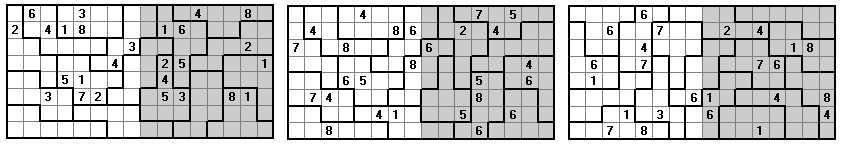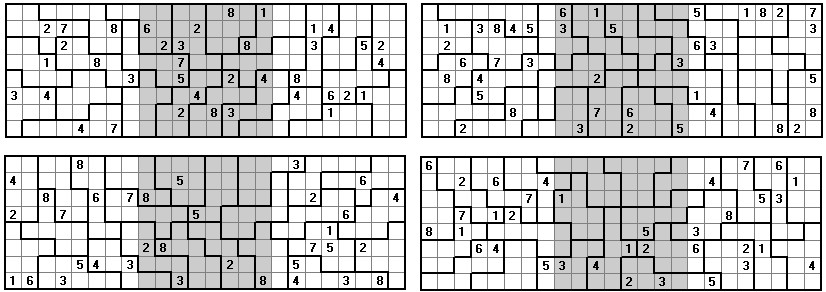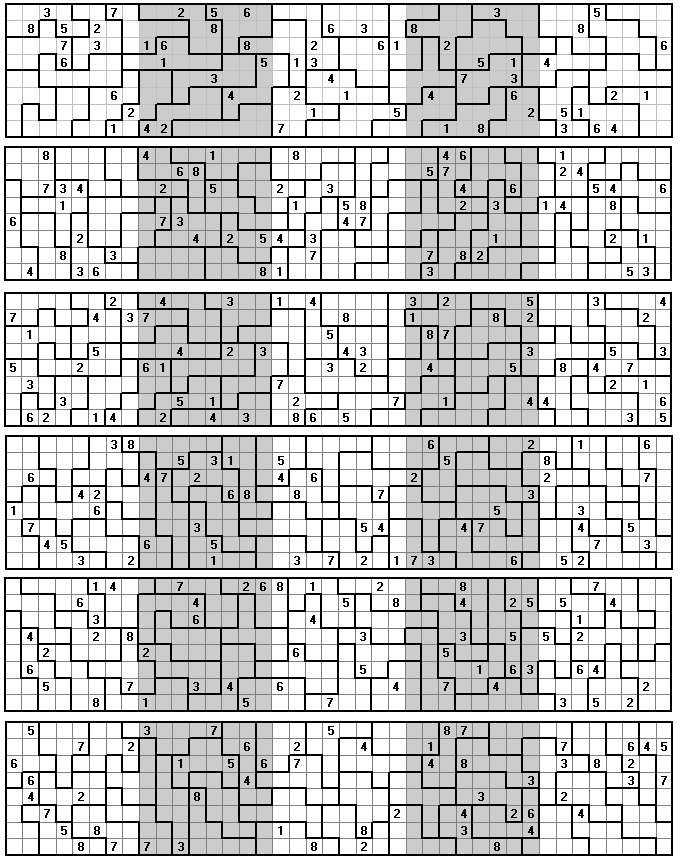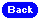Octomino Sudoku ProblemsTwo Square

These problems are based on constructions made from the set of 16 convex pieces we get if we add two squares to a 2x3 rectangle.Alternatively, we can use the set of convex pieces obtained by adding two parallel dominoes to a 2x2 square.Three Square

If we add a 2x2 square to the tetrominoes we get a set of 24 pieces.Four Square

These problems are based on constructions made with a single polyomino.Five Square

Here we use the set of forty octominoes formed by adding two dominoes to a 2x2 square.